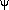### BCD Statistics R/Shiny Applications for Learning Statistical Concepts

Note that these applications are under regular development and expansion. Check back often.
These Shiny apps are developed by B. Dudek, Univeristy at Albany using the Shiny package from RStudio and R.
Eventually, the code for these apps will be shared within the apps.

Last updated 8-3-2022
These shiny apps may not work well with Internet Explorer or Edge at the moment. Use Firefox, Chrome, Safari.

Probability Distribution Apps (visualizations to supplement/replace textbook tables of these distributions)Binomial DistributionStandard Normal DistributionStudent's "t" DistributionChi-square DistributionF Distribution
Univariate and Bivariate Data and Computation VisualizationsUnivariate Description and GraphsVisualize Sum of Squares and VarianceVisualize Bivariate Correlation and Covariance - the Rectangles App.Simple Regression Simulation, - visualize predicted values and linear fitVisualize Components of LOESS Smoothing
Core Concepts Related to InferenceSampling Distributions and Central Limit Theorem VisualizationVisualize Type II Error Rates in a One-sample test of a MeanVisualize Simulated Distributions of p-values NEWDistribution Overlap and Effect Size Indices NEWVisualize Confidence Intervals of the MeanVisualize the relationships between confidence intervals and p-values
Other Miscl AppsMaximum Liklihood Basics demonstrated wtih the binomial distribution.Orthogonal Polynomials and "Trend Analysis" in an ANOVA contextVisualize interactions, simple main effects, simple slopes and model surfaces in 2-IV linear models